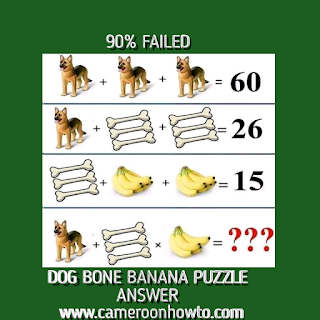# How do you solve math puzzles?Stay home and puzzle

Many people fail math puzzles not because they don't know math but because of some little anxiety, tricks in the puzzle and lack of basic basic Mathematics application. Today I will show you how to solve math puzzles to always get the correct answer, I will also solve a math puzzle for you to better understand.

## How to solve any math puzzles and get a correct answer

To solve any math puzzle correctly, apply the following tips below.

• Don't be to anxious

• Find the trick in it

• Apply mathematical rules

Get an explanation of the points above below.

Don't be too anxious: Any time you come across any math puzzle, take a deep breath and relax, apply what math says, not the shortest way you think at the moment. Also be careful to over relax because some puzzles are time based

Read all the puzzle: Before giving a fast answer to any math puzzle, Make sure to read it carefully to the end first because in most cases, the tricks are always toward the end of the puzzle. When you read it, you will easily Identify the trick.

Find the trick: Of course When reading a puzzle to the end, you are finding the trick in it. Immediately you find all the tricks, start solving.

Apply mathematical rules: Yes you hear math puzzles, not English puzzles. When it's a math puzzle, you only need to apply math rules like BODMAS, PEMDAS etc. It's Also important to note that all math puzzles come from Mathematics topics like Simultaneous equations, Making subject of the formlar etc. So, when you come across any math puzzle, to get the correct answer, try to identify the topics from math in the puzzles.

## Example math puzzle

### Answer to dog bone banana math puzzle# dog bone banana math puzzle

Dog + bone × bananas = ?

The correct answer would be 32 not 92, the trick in the math Puzzle is found in the last equation because the bunch of bananas has four bananas meanwhile that in the third equation has 5 bananas. Another trick is to multiply before adding.

Many game lovers like solving math puzzles suduku, quizzes like this one to refresh their brain.

Here's the puzzle extraction:

If

Dog + dog + dog  = 60,

Dog + 3bones + 3bones = 26

Bones +5 bananas + 5 bananas = 15

then,

Dog + bones × four bananas = ?

I will form four equations, solve equation 1, 2 and three mathematically and then substitute answers in the final equation and simplify.

Equation one

3dogs =60

Implies dog   = 60÷3,

Dog = 20.

Equation two

20 + 6bones = 26

Implies 6bones = 26 minus 20

6bones = 6

Bone = 6 ÷ 6

Bone  = 1

Equation three

3 + 6 banana + 6 banana = 15,

12 banana = 15 minus 3

12 banana = 12

Banana = 12/12=  1.

Therefore, the value of Dog Bone and banana are as Thus:

Dog = 20

Bone = 1

Banana = 1

Remember you can still get all the values by simply counting but it's important to use math rules in case of larger numbers.

Final equation

I will substitute the values of the variables above in the final equation below and simplify.

Dog + 3 bone × 4 bananas = ?

20 + 3 × 4 = ? ( Apply BODMAS)

20 + 12= ?

32 = ?

So the correct answer to the tricky  math Puzzle "dog + 3bones × 4 bananas =?"  Is

= 32.

If you don't apply BODMAS, you will get 92 which is a mathematically wrong answer.

## Conclusion

If you follow all the tips above and also apply them as I did in the example above, you will always solve and get all your math puzzle answers correct.